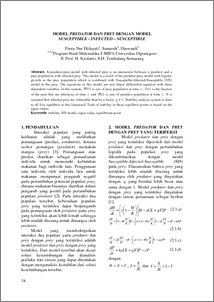# MODEL PREDATOR DAN PREY DENGAN MODEL SUSCEPTIBLE - INFECTED – SUSCEPTIBLE

Hidayati, Firsty nur and Sunarsih, Sunarsih and Djuwandi, Djuwandi (2010) MODEL PREDATOR DAN PREY DENGAN MODEL SUSCEPTIBLE - INFECTED – SUSCEPTIBLE. Jurnal Matematika, 13 (1). pp. 34-39. ISSN 1410-8518Preview
PDF
81Kb

## Abstract

A predator-prey model with infected prey is an interaction between a predator and a prey population with infected prey. This model is a result of the predator-prey model with logistic growth in the prey population which is combined with Susceptible-Infected-Susceptible (SIS) model in the prey. The equations in this model are non linear differential equation with three dependent variables. In this system, is size of prey population at time , is the fraction of the prey that are infectious at time and is size of predator population at time . It is assumed that infected prey are vulnerable than by a factor . Stability analysis system is done to all five equilibriain this linearized. Each of stability in those equilibria points is based on theeigen values.

Item Type: Article Q Science > QA Mathematics Faculty of Science and Mathematics > Department of Mathematics 26876 INVALID USER 19 Apr 2011 16:09 19 Apr 2011 16:09

Repository Staff Only: item control page